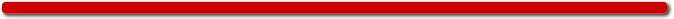Holiday Caching Game

In an effort to create a Holiday goodie that all can enjoy, we developed a Holiday Caching Game with a bit of a twist. This game contains 12 boxes with sections for all grade levels (7-12).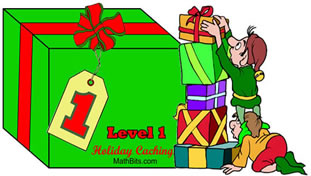Boxes 1 - 3 Level 1 - Basic Math Student Worksheet Topics: ratio, percentages, area, perimeter, circumference, Pythagorean Theorem, logical reasoning. Word problems.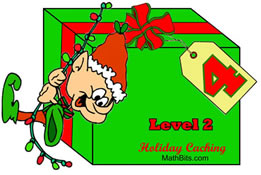Boxes 4 - 6 Level 2 - Algebra 1 Student Worksheet Topics: algebraic expressions, area, perimeter, mean, solving linear equations, circumference, quadratic equations, exponents, order of operations, logical reasoning. Word problems.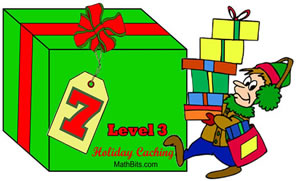Boxes 7 - 9 Level 3 - Geometry Student Worksheet Topics: types of angles and triangles, Pythagorean Theorem, parallel lines and angles, congruent triangles, quadratic equations,logical reasoning. Word problems.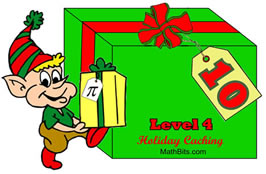Boxes 10 - 12 Level 4 - Algebra 2 Student Worksheet Topics: functions, basic trigonometry, exponential expressions, linear quadratic systems, inequalities, polynomials, equations of circles, area of circles, logical reasoning. Word problems.

A CERTIFICATE is available at the end of each Level.

Teachers can assign ONLY the Boxes that apply directly to their grade level, or, they can assign ALL of the Boxes leading up to their grade level.

We tried to keep the topics appropriate for a class that has only been in session a little over 3 months. Your Level may see problems that were developed in the later part of the previous grade level.